### Could someone please explain this code to me?

1397   6   0Member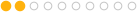30 posts
Joined: Nov. 2017
Offline
Hey guys! I'm following this applied houdini tutorial
https://vimeo.com/263107417 [vimeo.com]

I got to a point where, we're trying to ray the points from the target, and the source so we can get the lightning to go from the aforementioned source, to the target. To do that

We're making the normals of the targets point towards the source of the ligtning, but subracting the position of the points of the ray node, from the position of the targets them selves. It looks like thisSo it's working. However, right before this, the code looked like this

@N = point(1,"p", @ptnum) - @P;

and it looked like this.I changed the "p" to a "P", and that fixed it.

What i'm trying to figure out is, what is the difference between "p" and "P". In the tutorial he doesnt say to do that, so that's why it took me so long to figure out.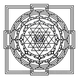Member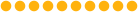1859 posts
Joined: Sept. 2015
Offline
This difference is that capital P actually refers to an existing attribute which are the points positions.

In this case lower p is not referring to anything at all, unless it was created explicity somewhere else up the chain.

Him having lower p was just probably a typo mistake he missed.Member30 posts
Joined: Nov. 2017
Offline
BabaJ
This difference is that capital P actually refers to an existing attribute which are the points positions.

In this case lower p is not referring to anything at all, unless it was created explicity somewhere else up the chain.

Him having lower p was just probably a typo mistake he missed.

Wow thank you! That makes perfect sense. I'm still slowly learning, but its' getting there.Member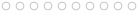1 posts
Joined: Oct. 2020
Offline
Hello, i am newbie to Houdini, i am watching the applied Houdini tutorial particle 3 as well, and i encountered this code. but i cannot get my head around.

@N = point (1, "P", @ptnum)- @P;

what does this code even mean.
i simply don't understand what is point (1, "P", @ptnum), it doesn't make sense. point is definitely not @P, it looks like a local variable, and what is the stuff in the bracket.
usually P would get followed by (x, y, z) but here 1 is not value 1 but the input.

can somebody point me to the right direction please.Member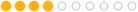94 posts
Joined: March 2016
Offline
The function
```point(1,'P',@ptnum)
```
returns the point position for the same point number coming in via slot one of the wrangle (keep in mind they count up 0,1,2,3). You are then subtracting the current point position
```@P
```
and the storing that value as your point normal.
Edited by Hatchery - June 16, 2022 11:13:54
Love HoudiniMember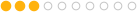74 posts
Joined: July 2019
Online
gimletful
Hello, i am newbie to Houdini, i am watching the applied Houdini tutorial particle 3 as well, and i encountered this code. but i cannot get my head around.

@N = point (1, "P", @ptnum)- @P;

what does this code even mean.
i simply don't understand what is point (1, "P", @ptnum), it doesn't make sense. point is definitely not @P, it looks like a local variable, and what is the stuff in the bracket.
usually P would get followed by (x, y, z) but here 1 is not value 1 but the input.

can somebody point me to the right direction please.

Letters predefined by @ are attributes, you can find their values in Geometry Spreadsheet tab. In some cases, you may want to read attributes from another geometry, like here, from geometry connected to second input of attribute wrangle (All inputs have numbers starting from 0). @ can't read attributes from inputs other than 0, so you need special VEX function to read the attribute. You want to read Position attribute of current point from second input, so you need:
Point function, (there are also vertex(), prim(), detail() for attributes stored on vertices, primitives, details geometry components)
(
1, - input number (to read attribute from second input of attribute wrangle node)
"P", - attribute's name you want to read (N(normals),P(positon),"v"(velocity),"Cd"(color) etc.)
@ptnum - special type of attributes, contains number of current point(there are also @primnum(current primitive), @vtxnum( cur.vertex)
)
Attribute wrangle will pass over every point and calculate the code
@N = point (1, "P", @ptnum)- @P;
Mathematically the code means:
Attribute wrangle(repeat calculations for every point from first(0) input):
Normal vector (x,y,z) = Second geometry's point position vector (x,y,z) - First geometry's point position vector (x,y,z)

PS:
Theoretically you can replace it with:
vector Normal = point(1, "P", @ptnum) - point(0, "P", @ptnum); //Vector variable Normal equal to vector position of point from first input minus vector position of point from second input)
setpointattrib(0, "N", @ptnum, N); //replace normal attribute with Normal variable.
But there is no need to make it so complicated, @ allows you read and write attributes freely but only for components from first(0) input.

Simple tutorials about geometry components and math operations in 3d space [www.sidefx.com]

Geometry attributes documentation [www.sidefx.com]
VEX functions reference list [www.sidefx.com]
Edited by Faitel - June 16, 2022 12:05:27Member6595 posts
Joined: July 2007
Offline
you can also use:
```v@N = v@opinput1_P - v@P;
```

since v@opinput1_P is essentially the same as point(1, "P", @ptnum) the same way as v@P is the same as point(0, "P", @ptnum)
Tomas Slancik
FX Supervisor
Method Studios, NY